{

# Does Fitness Depend on Size?

W. B. Langdon
School of Computer Science,
The University of Birmingham, UK

14 July 1998

## Does Fitness Depend on Length?

• explicitly assumes fitness distribution of 3-input Boolean functions is independent of program size, cf. Fig. 1. Figure 2 shows this is true.

• Figures 2 to 9 show fitness distribution is more or less independent of program length once a certain minimum size is exceeded in a range of discrete problems.

• A limit can be proved for the special case of the parity problems with one function

• However symmetric full trees need not be typical of the asymmetric trees which form most of the search space.

Monte Carlo sampling code and tree counting code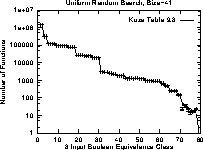Figure 1:   Number of functions of length 41 in each equivalence class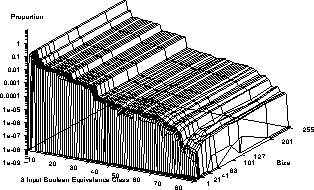Figure 2:    Proportion of functions in each equivalence class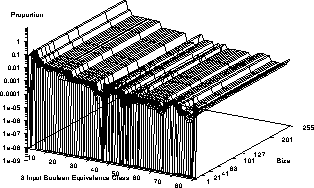Figure 3:   Proportion of functions in each equivalence class with XOR in function set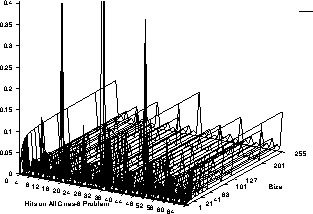Figure 4:   Number of ones returned by 6-input Boolean functions (note linear scale)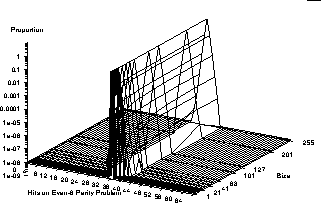Figure 5:   Even-6 parity program space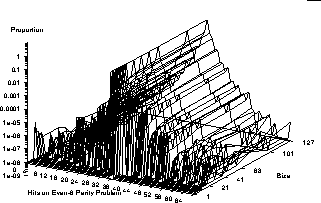Figure 6:   Even-6 parity program space, including XOR in function set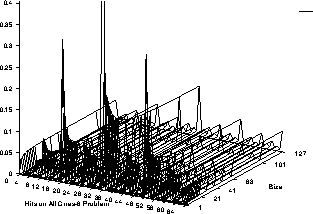Figure 7:   Number of ones returned by 6-input Boolean functions, XOR included (note linear scale)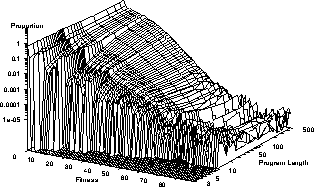Figure 8:   Proportion of Ant programs of a given length by their fitness. Values for lengths 15 and above are based on Monte Carlo sampling.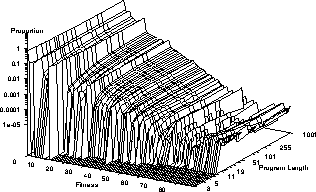Figure 9:    Proportion of Ant programs of a given length by their fitness. Using binary function set.

William B Langdon
Wed Jul 29 14:55:34 BST 1998